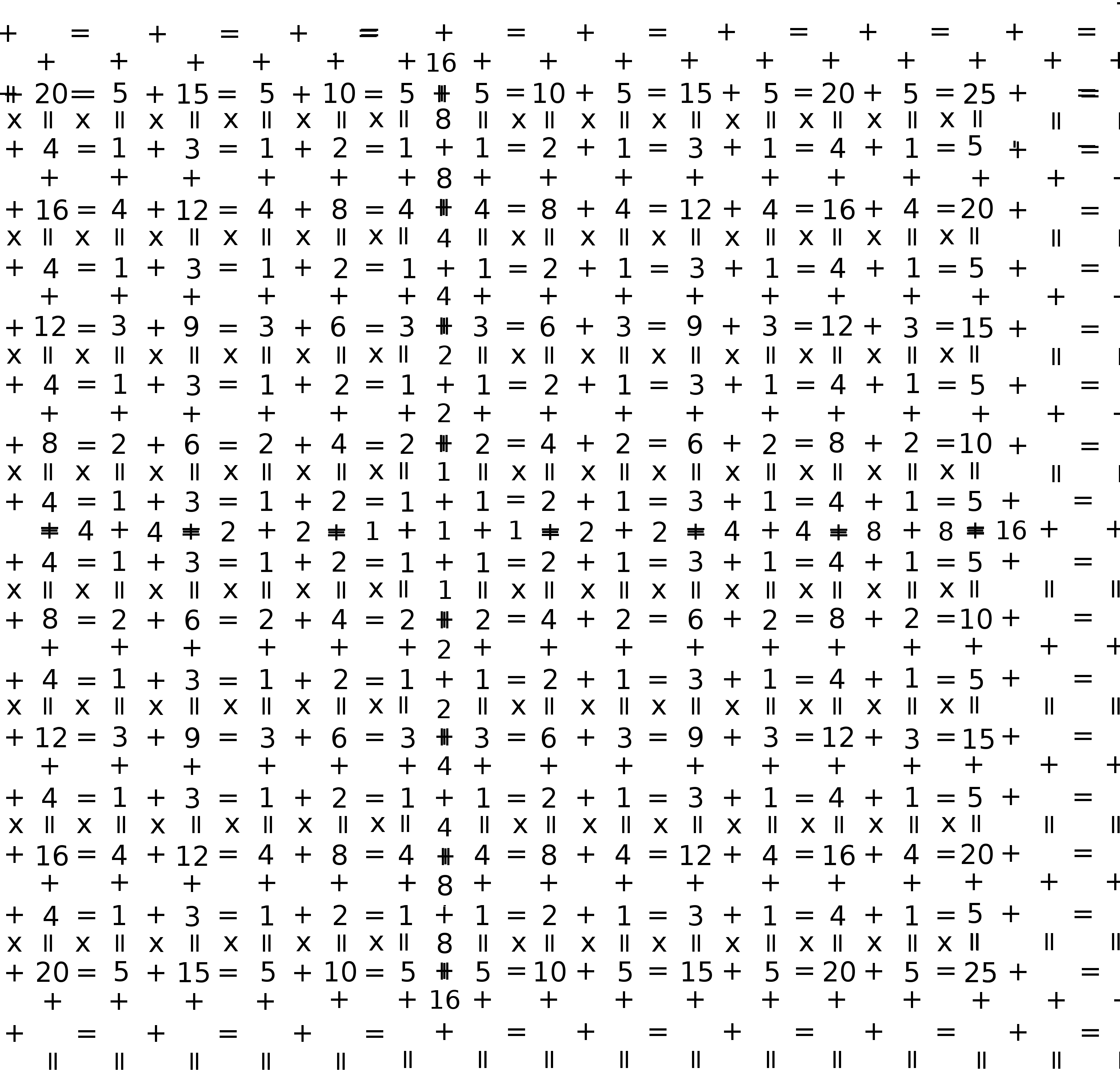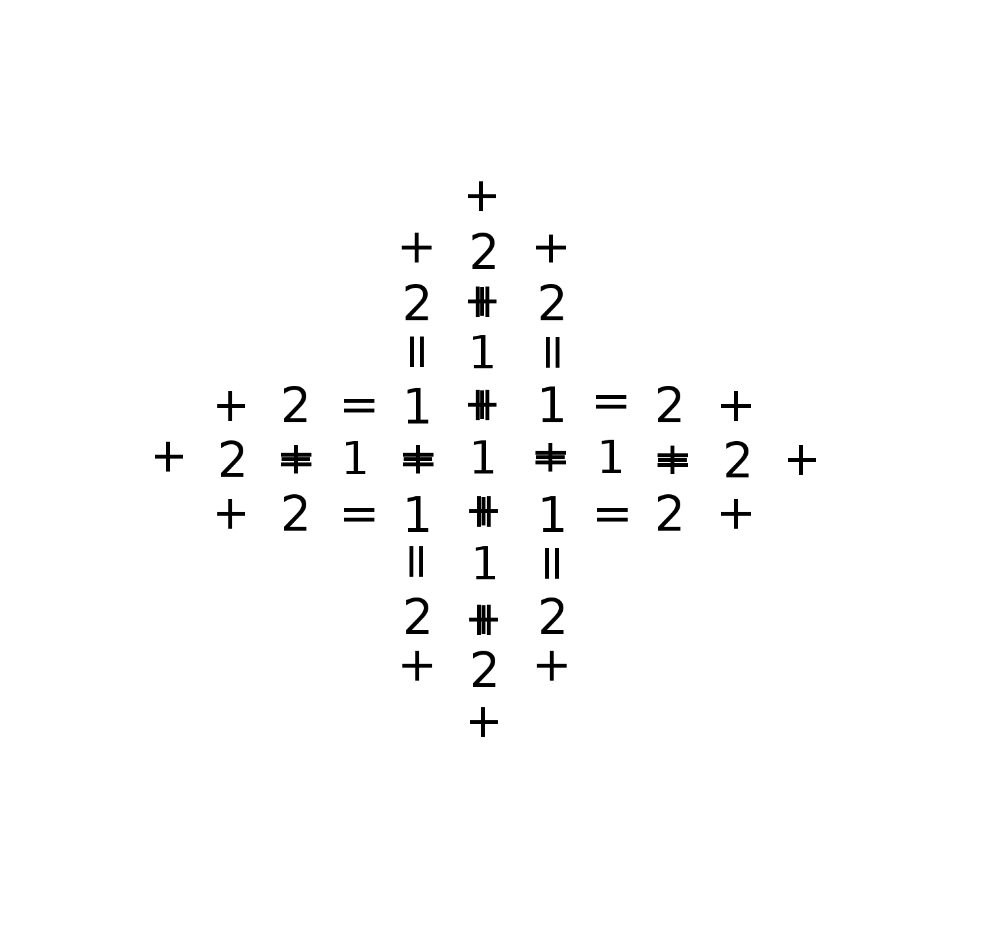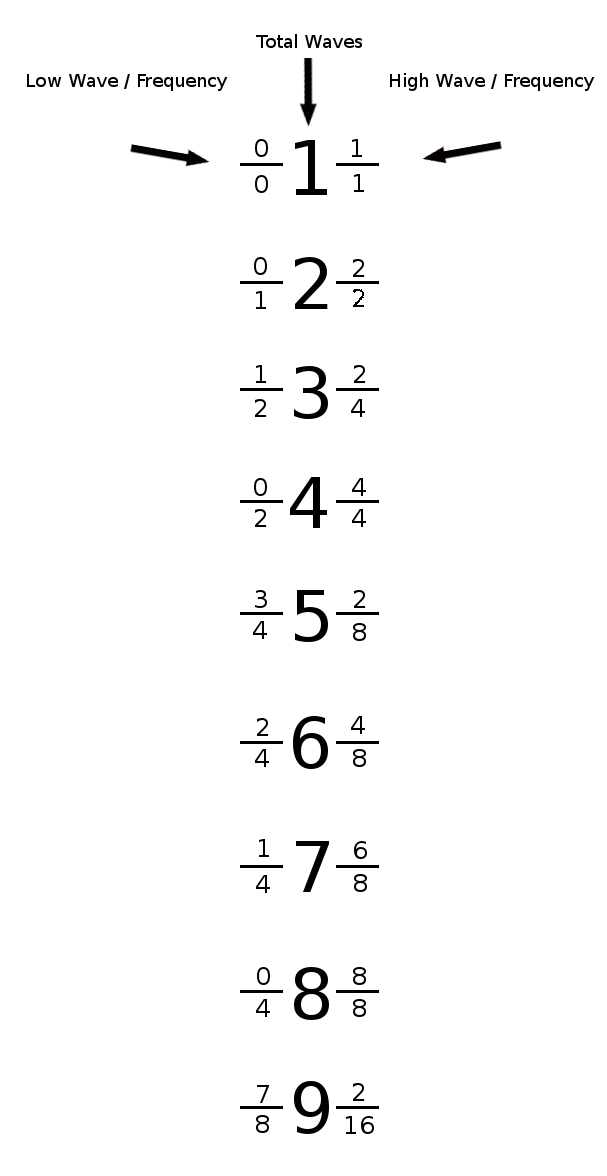# Is Fractal Binary a New Math

#### ashesmi

##### New member
Here is Dr Jonathan Tallant a famous math phlosopher

Fractal Binary is a type of math that uses waves in performing it's calculations. Frequency Bits, (Numbers), are generated as waves. These positive and negative waves are then added and subtracted together 'at the same time', waves have a tendency to do that.

Many Calculations occur inside of a Single Calculation.

Here is New Math http://ashesmi.yolasite.com/fractal-binary.php

And has anyone here seen this Matrix Before?Where is this Matrix in history? Refrences?

#### Sudharaka

##### Well-known member
MHB Math Helper
Hi everyone,I think that this should be moved to the Chat room. The other topics sub-forum is dedicated to mathematical questions about Physics, Biology etc. However the thread in question provokes discussion rather than a specific math question. Please let me know if you think otherwise.#### ashesmi

##### New member
Hi everyone,I too think that this should be moved to the Chat room. The other topics sub-forum is dedicated to mathematical questions about Physics, Biology etc. However the thread in question provokes discussion rather than a specific math question. Please let me know if you think otherwise.I am good with whatever you guys want to do, heads up though, I JUST Solved for (Y and Z) from only knowing (X) (so long as Y and Z together are not Prime Numbers) in the Equation (X = Y + Z)....ahhhh I think I need to talk to someone about this....Let me just verify this...a little later on, my head is spinning.

Please let me know what you all decide to do with this topic, I thank you for looking at it and if it is in coffee room please let me know, I am new here and I would like to get to know the people#### ashesmi

##### New member
Hi everyone,I think that this should be moved to the Chat room. The other topics sub-forum is dedicated to mathematical questions about Physics, Biology etc. However the thread in question provokes discussion rather than a specific math question. Please let me know if you think otherwise.Hey Sudharaka want to check this out? The Funny Symbols are ( + , = ) signs, in one direction they add in the other direction they equil. Have You Seen these Symbols before? and Have You see this Matrix Before?It is Near Complete! Hey Sudharaka, I got question can I ask them here in the physics forum about the Math Equation here to describe the Series from the Video Here?

Here is the Equation to Describe the wave series and it works!I can Do Logs using Waves / Frequency. I do not need a calcuator or log tables or slide ruler. The Math works Have you Ever see this Physics Math?

I can solve for 124,543,342,232,122,123 without using a calcuator, slide ruler or Log tables

Just 3 quesions for you...

1. Can You solve for 124,543,342,232,122,123 without using a calcuator, slide ruler or Log tables?

2. Have You Seen these Symbols before?

3. Have You see this Matrix Before?

Can You Help me find someone to work with on this?

Last edited:

#### topsquark

##### Well-known member
MHB Math Helper
I really have no idea what you are talking about. Your video implies this to be merely a breakdown of a system into a set of harmonics so I don't see how this has any new application to Physics.

Also, are you aware that step 2 gives an equation that says $$T' \lambda = 0$$? That sort of knocks out many of your definitions and gives a simpler set of equations relating to your variables. Perhaps simpler to an extreme that you don't want.

-Dan

#### Bacterius

##### Well-known member
MHB Math Helper
Well I've seen multiplication done through a Fast Fourier Transform (which involves wave superposition) but I've never heard of this kind of math before.

#### Sudharaka

##### Well-known member
MHB Math Helper
Hey Sudharaka want to check this out? The Funny Symbols are ( + , = ) signs, in one direction they add in the other direction they equil. Have You Seen these Symbols before? and Have You see this Matrix Before?
Hi again,

I am sorry, but I know nothing about the kind of math you are speaking about. Perhaps other members will have the knowledge to answer your questions and I hope you'll find your answers soon.#### ashesmi

##### New member
Well I've seen multiplication done through a Fast Fourier Transform (which involves wave superposition) but I've never heard of this kind of math before.
If I want to add Waves in Fractal Binary in Parallel, The Numbers (1, 2, 3, 4) are collided with each other at the same time like waves in nature. We don't have to Add the Results to the next number, all of the numbers together are the result. This way Many Calcuations occure in side of a Simgle Calcuation.

I have heard of Fourier Transformations before, I am not an Expert, they are Waves, but these Waves behave like the waves we see in the Nature that exaists around us in this Level of Existance, correct? These waves behave like the waves we see in water? If so, there would be 1 wave, then to 2 equal waves, then 3 equal waves, then 4 equal waves and so on and so on used in many of his calcuations?

With Fracal Binary it appears that as the Wavelength Split's in Half, the Wavelengths are not always equil in size. When we get to the number 3, their are NOT 3 Equil Wave Lengths, instead their are 2 Different Wavelenght Sizes, but their are 3 Wavelengths.

There would be 1 wave, (1/2) the origonal Wave Lenght, and 2 Waves, 1/4 the Origonal Wave Length. The Amplitudes in Generating the Frequancy Bits are always the same, but the Frequency's can become higher.

As the Number increases in size, there are more wavelengths inside of it. The Number becomes more Energitic, Heaver or Faster.

I am working on adding them in Series and Parellel. If I were to add, 1 plus 2 plus 3 plus 4 with todays computing, I would add 1 to the number 2, then the result would be added to 3, then the result would be added to 4. This took 3 Calcuations.

If I want to add Waves in Fractal Binary in Parallel, numbers (1, 2, 3, 4) are collided with each other at the same time, like waves in nature. We don't have to Add the Results to the next number, all of the numbers together ARE the result. This way Many Calcuations occure in side of a Simgle Calcuation. I am guessing that the Amplitude can be allowed to change when adding the Frequncy Bits together? I am experimenting with differnt methods, some with Allowing the Amplitude and Frequency that Exist inside of one another to change, this way they are Louder (Amplitude) and / or Faster (Frequency).

Other methods are by keeping the Amplitude the same, but squish the Frequencys...other methods are allowing the Lenght of the number to become bigger, and I am sure other methods can be tried as well. Can You (or anyone here) think of any?

Today if we have the number 10 we call (X) and we want to know what 2 numbers, we call (Y + Z), were used in it's genertation by Addition, it is impossible to do (to the best of my knowledge), if we know what X is. But using Fractal Binary I think it may be possible to discover where 10 came from, because every number is unique, they are all from '1' so 10 could be described to us in many different ways in Fractal Binary.

10 could be represented as 1 + 9, 2 + 8, 3 + 4 + 6, 5 + 5, 6 + 4, 7 + 3, 8 + 2, 9 + 1...This is the Number that would be stored and later given to us to solve. We would just somehow play the equation backwards, (still working on that, trying to wrap my mind around this fractal thinking is sureal)

With Fractal Binary, 10 would be expressed as (Y + Z)? Zen thinking

Finally if the origonal number, 1, is 1 wave length over 1 Frequency and the Origonal Frequency is 1 Wave / Second (second is only an example)...the number 1 would take 1 second to count, the number 10000000 would have 10000000 wavelengths inside of the Same Bit size, as the Number 1, but these 10000000 wavelengths would also take 1 second as well. This is in Generating the Pure Fractal Wave / Frequency Bits

Here is the (1 + Matrix) I cal it the 2112 Pic, drew this in Grade school, this type of fractal recursion math was instinctive to me, I am sure many other kids doodles this Matrix Below as well...

Did you check out the 2112 Matrix? It is Actually the (1+Matrix) but I am a bit of a (Rush) Fan and the 2112 pattern is NeatThis is the Matrix that the wave over frequency bits would reside in, I am guessing? Below is a 2 Based Matrix, there are different Bases I am experimenting as well. This is like a Fractal Version of the Cartesian Plane (Not really, but it is a Number Plane, a Matrix)....The opposite Plane (Matrix) would be the ( - 1 Matrix ), it is not displayed here, these Frequency bits are Negative waves when we collide the negative numbers with Positive numbers they just cancel each other out instainiously.And this is How the Pure Fractal Binary Bits existing in the Matrix Above would be displayed to Humans...I am trying to Add 2 of the Numbers above in Parallel on Paper, and try to find a way to take the result of whatever those 2 numbers were when added so I can find the origonal numbers in its generation...Do You Follow?

Last edited:

#### topsquark

##### Well-known member
MHB Math Helper
...Do You Follow?
No.

Can you do a simple example point by point and explain just what the heck "total physical wave sequence," etc. are and how they relate to the problem. In fact, start with explaining what the problem is in the first place.

-Dan